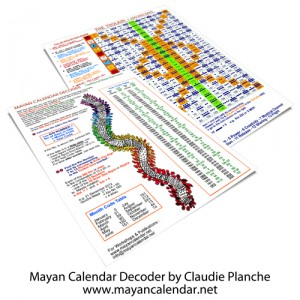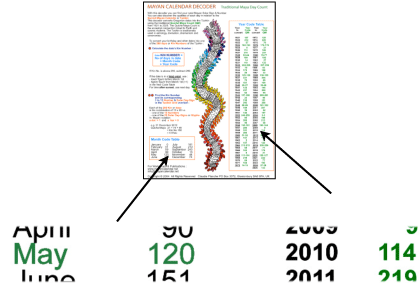# How To Use the Mayan Calendar DecoderMayan Calendar Decoder

In order to convert a birthday or another date into the Tzolkin Mayan Calendar, we designed and created our own ‘Decoder’ based on the traditional day count. This count has been kept by the Quiché Maya from Guatemala since the Classic Period.

Using the Decoder tables it only takes two sums to find the equivalent Tzolkin date using the formula :Formula To Calculate Equivalent Tzolkin Date

If the time is after local sunset, take the next day.

Example
Let’s convert the 27th May 2010 before sunset.

Decoder Page 1
Referring to the Month Code Table and the Year Code Table we apply the formula above. Please note that for leap years you take the higher figure from 1st March (+ 1 day).

Kin Number = 27 + 120 + 114 = 261Decoder Page 1

If the sum is more than 260 (the number of the Tzolkin cycle of 260 days) we simply substract 260. In our example it is therefore :
Kin Number = 261 – 260 = 1

This means that our date is the number 1 or first day in the grid of 260.

So May 27th 2010 is the beginning of another Tzolkin cycle lasting about 9 months. At this turning point we can review the previous cycle and prepare for the next gestation!

Decoder Page 2
Using the Tzolkin grid, we then find the Mayan Solar Sign and Number between 1 and 13 in a dot bar notation. Remember the Mayan notation :

Our example is very straight forward as 1 IMIX (pronounced ‘imish’) is exactly at the beginning on the left hand corner.Decoder Page 2

You will soon get used to the Tzolkin Mayan Calendar and easily convert dates with our Mayan Calendar Decoder.

Click here to order our Mayan Calendar Decoder as part of our Mayan Calendar kit